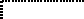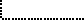Common Lisp the Language, 2nd EditionNext: Primitive Hash Function Up: Hash Tables Previous: Hash Tables

# 16.1. Hash Table Functions

This section documents the functions for hash tables, which use objects as keys and associate other objects with them.

[Function]
make-hash-table &key :test :size :rehash-size :rehash-threshold

This function creates and returns a new hash table. The :test argument determines how keys are compared; it must be one of the three values #'eq, #'eql, or #'equal, or one of the three symbols eq, eql, or equal. If no test is specified, eql is assumed.X3J13 voted in January 1989 (HASH-TABLE-TESTS)   to add a fourth type of hash table: the value of #'equalp and the symbol equalp are to be additional valid possibilities for the :test argument.

Note that one consequence of the vote to change the rules of floating-point contagion (CONTAGION-ON-NUMERICAL-COMPARISONS)   (described in section 12.1) is to require =, and therefore also equalp, to compare the values of numbers exactly and not approximately, making equalp a true equivalence relation on numbers.

Another valuable use of equalp hash tables is case-insensitive comparison of keys that are strings.The :size argument sets the initial size of the hash table, in entries. (The actual size may be rounded up from the size you specify to the next ``good'' size, for example to make it a prime number.) You won't necessarily be able to store precisely this many entries into the table before it overflows and becomes bigger, but this argument does serve as a hint to the implementation of approximately how many entries you intend to store.X3J13 voted in January 1989 (ARGUMENTS-UNDERSPECIFIED)   to clarify that the :size argument must be a non-negative integer.

X3J13 voted in June 1989 (HASH-TABLE-SIZE)   to regard the preceding description of the :size argument as definitive: it is approximately the number of entries that can be inserted without having to enlarge the hash table.The :rehash-size argument specifies how much to increase the size of the hash table when it becomes full. This can be an integer greater than zero, which is the number of entries to add, or it can be a floating-point number greater than 1, which is the ratio of the new size to the old size. The default value for this argument is implementation-dependent.The :rehash-threshold argument specifies how full the hash table can get before it must grow. This can be an integer greater than zero and less than the :rehash-size (in which case it will be scaled whenever the table is grown), or it can be a floating-point number between zero and 1. The default value for this argument is implementation-dependent.X3J13 voted in June 1989 (HASH-TABLE-SIZE)   to replace the preceding specification of the :rehash-threshold argument with the following: The :rehash-threshold argument specifies how full the hash table can get before it must grow. It may be any real number between 0 and 1, inclusive. It indicates the maximum desired level of hash table occupancy. An implementation is permitted to ignore this argument. The default value for this argument is implementation-dependent.An example of the use of make-hash-table:

```(make-hash-table :rehash-size 1.5
:size (* number-of-widgets 43))
```

[Function]
hash-table-p object

hash-table-p is true if its argument is a hash table, and otherwise is false.

```(hash-table-p x) == (typep x 'hash-table)
```

[Function]
gethash key hash-table &optional default

gethash finds the entry in hash-table whose key is key and returns the associated value. If there is no such entry, gethash returns default, which is nil if not specified.

gethash actually returns two values, the second being a predicate value that is true if an entry was found, and false if no entry was found.

setf may be used with gethash to make new entries in a hash table. If an entry with the specified key already exists, it is removed before the new entry is added. The default argument may be specified to gethash in this context; it is ignored by setf but may be useful in such macros as incf that are related to setf:

```(incf (gethash a-key table 0))
```

means approximately the same as

```(setf (gethash a-key table 0)
(+ (gethash a-key table 0) 1))
```

which in turn would be treated as simply

```(setf (gethash a-key table)
(+ (gethash a-key table 0) 1))
```

[Function]
remhash key hash-table

remhash removes any entry for key in hash-table. This is a predicate that is true if there was an entry or false if there was not.

[Function]
maphash function hash-table

For each entry in hash-table, maphash calls function on two arguments: the key of the entry and the value of the entry; maphash then returns nil. If entries are added to or deleted from the hash table while a maphash is in progress, the results are unpredictable, with one exception: if the function calls remhash to remove the entry currently being processed by the function, or performs a setf of gethash on that entry to change the associated value, then those operations will have the intended effect. For example:

```;;; Alter every entry in MY-HASH-TABLE, replacing the value with
;;; its square root.  Entries with negative values are removed.
(maphash #'(lambda (key val)
(if (minusp val)
(remhash key my-hash-table)
(setf (gethash key my-hash-table) (sqrt val))))
my-hash-table)
```X3J13 voted in January 1989 (MAPPING-DESTRUCTIVE-INTERACTION)   to restrict user side effects; see section 7.9.[Function]
clrhash hash-table

This removes all the entries from hash-table and returns the hash table itself.

[Function]
hash-table-count hash-table

This returns the number of entries in the hash-table. When a hash table is first created or has been cleared, the number of entries is zero.[Macro]
with-hash-table-iterator (mname hash-table) {form}*

X3J13 voted in January 1989 (HASH-TABLE-PACKAGE-GENERATORS)   to add the macro with-hash-table-iterator.

The name mname is bound and defined as if by macrolet, with the body forms as its lexical scope, to be a ``generator macro'' such that successive invocations (mname) will return entries, one by one, from the hash table that is the value of the expression hash-table (which is evaluated exactly once).

At each invocation of the generator macro, there are two possibilities. If there is yet another unprocessed entry in the hash table, then three values are returned: t, the key of the hash table entry, and the associated value of the hash table entry. On the other hand, if there are no more unprocessed entries in the hash table, then one value is returned: nil.

The implicit interior state of the iteration over the hash table entries has dynamic extent. While the name mname has lexical scope, it is an error to invoke the generator macro once the with-hash-table-iterator form has been exited.

Invocations of with-hash-table-iterator and related macros may be nested, and the generator macro of an outer invocation may be called from within an inner invocation (assuming that its name is visible or otherwise made available).

X3J13 voted in January 1989 (MAPPING-DESTRUCTIVE-INTERACTION)   to restrict user side effects; see section 7.9.

Rationale: This facility is a bit more flexible than maphash. It makes possible a portable and efficient implementation of loop clauses for iterating over hash tables (see chapter 26).

```(setq turtles (make-hash-table :size 9 :test 'eq))
(setf (gethash 'howard-kaylan turtles) '(musician lead-singer))
(setf (gethash 'john-barbata turtles) '(musician drummer))
(setf (gethash 'leonardo turtles) '(ninja leader blue))
(setf (gethash 'donatello turtles) '(ninja machines purple))
(setf (gethash 'al-nichol turtles) '(musician guitarist))
(setf (gethash 'mark-volman turtles) '(musician great-hair))
(setf (gethash 'raphael turtles) '(ninja cool rude red))
(setf (gethash 'michaelangelo turtles) '(ninja party-dude orange))
(setf (gethash 'jim-pons turtles) '(musician bassist))

(with-hash-table-iterator (get-turtle turtles)
(labels ((try (got-one &optional key value)
(when got-one      ;Remember, keys may show up in any order
(when (eq (first value) 'ninja)
(format t "~%~:(~A~): ~{~A~^, ~}"
key (rest value)))
(multiple-value-call #'try (get-turtle)))))
(multiple-value-call #'try (get-turtle))))     ;Prints 4 lines
Michaelangelo: PARTY-DUDE, ORANGE
Raphael: COOL, RUDE, RED
Donatello: MACHINES, PURPLE
=> nil
```[Function]
hash-table-rehash-size hash-table
hash-table-rehash-threshold hash-table
hash-table-size hash-table
hash-table-test hash-table

X3J13 voted in March 1989 (HASH-TABLE-ACCESS)   to add four accessor functions that return values suitable for use in a call to make-hash-table in order to produce a new hash table with state corresponding to the current state of the argument hash table.

hash-table-rehash-size returns the current rehash size of a hash table.

hash-table-rehash-threshold returns the current rehash threshold.

hash-table-size returns the current size of a hash table.

hash-table-test returns the test used for comparing keys. If the test is one of the standard test functions, then the result will always be a symbol, even if the function itself was specified when the hash-table was created. For example:

```(hash-table-test (make-hash-table :test #'equal)) => equal
```

Implementations that extend make-hash-table by providing additional possibilities for the :test argument may determine how the value returned by hash-table-test is related to such additional tests.Next: Primitive Hash Function Up: Hash Tables Previous: Hash Tables

AI.Repository@cs.cmu.edu